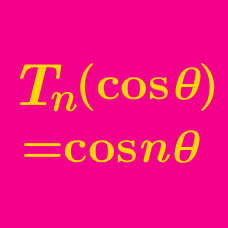Algebra

# Chebyshev Polynomials: Level 4 Challenges

Let $T_n$ be the nth Chebyshev Polynomial.

If $T_{90}(x)=\frac{\sqrt3}{2}$, then find the value of $T_{360}(x)$.

$\sum_{k \in S} \cos\left(\frac{2k\pi}{11}\right)$

Find the value of the summation above where $S = \{ 1,2,3,5,7,11 \}$: all the prime numbers between 2 and 11, together with 1.

$\large \displaystyle \prod_{n=1}^{2016} \sec\left(\dfrac{n\pi}{2017}\right)$

Evaluate the product above.

Given that the above is equal to $a^b$ where a is a prime number, find $a+b$.

Inspired by this problem when I solved it in 3 steps... All of which were wrong, but somehow I got the right answer.

$\prod_{k=1}^{n} \left(1 + 2\cos\frac{2k\pi}n\right)$

If $n$ is a prime number larger than 3, then find the value of the expression above.

Evaluate

$\displaystyle \sum_{k=1}^{45} \csc^2(2k-1)$

The angle is given in degrees.

×